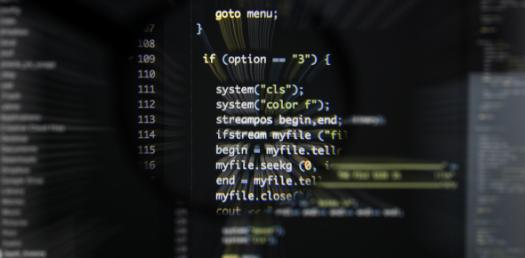# Intro To C Programming Quiz 2

11 Questions | Attempts: 1437
ShareSettingsThis is the second Quiz.

• 1.
The body of a while loop may never get executed.
• A.

True

• B.

False

• 2.
Int a = 0;numbers[ ] = {1, 3, 5, 7, 9, 13, 15, 27, 23}while (a < 5){        printf("%d", numbers[a]);}
• A.

1, 3, 5, 7, 9, 13, 15, 27, 23

• B.

The value of 1 will be output infinitely.

• C.

Nothing will output to the screen.

• D.

The value of 3 will be output infinitely.

• 3.
The following statement illustrates the use of what type of variable? (Hint: What is the total_sum variable called?)total_sum = total_sum + number;
• A.

Counter

• B.

Loop control

• C.

Initializer

• D.

Accumulator

• 4.
A program prompts the user for a value until the user enters a -1.  What kind of looping structure is needed for this case?
• A.

An accumulator-controlled loop

• B.

A conditional loop

• C.

A counter-controlled loop

• D.

A sentinel-controlled loop

• 5.
Which type of loop always executes at least once?
• A.

Sentinel-controlled loop

• B.

For loop

• C.

Do-while loop

• D.

Counter-controlled loop

• 6.
The process of testing flow of control between a main function and its subordinate functions is called _______________________.
• A.

Bottom-up testing

• B.

System integration testing

• C.

Top-down testing

• D.

Unit testing

• 7.
The subscript of the first element of an array is 0.
• A.

True

• B.

False

• 8.
The NULL character is placed at the ______________ of a string.
• A.

Beginning

• B.

Middle

• C.

End

• 9.
The NULL character used in strings in C is represented by _________.
• A.

/*

• B.

/0

• C.

\0

• D.

*0

• E.

\$0

• 10.
All elements in a multidimensional array can have different data types.
• A.

True

• B.

False

• 11.
What is the correct syntax for declaring and initializing an array that contains the numbers 1.1, 3.1, 4.1, 5.1, and 6.1)?
• A.

Double digits  = {1.1, 3.1, 4.1, 5.1, 6.1};

• B.

Double digits = {1.1, 3.1, 4.1, 5.1, 6.1};

• C.

Int digits = {1.1, 3.1, 4.1, 5.1, 6.1};

• D.

Double digits [ ] = {1.1, 3.1, 4.1, 5.1, 6.1};

## Related TopicsBack to top
×

Wait!
Here's an interesting quiz for you.